# Index of CoincidenceGiven a text string, the index of coincidence IC or IOC, is the probability of two randomly selected letters being equal. The use of index of coincidence was first proposed by William F. Friedman in 1922 [FRIEDMAN1996, KULLBACK1976]. According to historian David Kahn [KAHN1967, page 176], ``Revierbank Publication No. 22, written in 1920, when Friedman was 28, must be regarded as the most important single publication in cryptography. It took the science into a new world.'' See Clark [CLARK1977] for more about Friedman's personal life. The following shows the cover of Riverbank Publication No. 22 [FRIEDMAN1996]. It was printed in France in 1922 to save cost.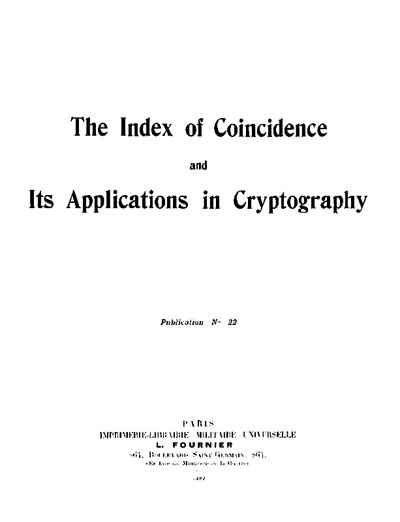Let the length of the text be N and let the size of the alphabet be n. Consider the i-th letter ai in the alphabet. Suppose ai appears in the given text Fi times. Since the number of ai's in the text is Fi, picking the first ai has Fi different choices and picking the second ai has only Fi-1 different choices because one ai has been selected. Since there are N(N-1) different ways of picking two characters from the text, the probability of having two ais is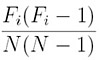Since the alphabet has n different letters and the above applies to each of them, the probability of having two identical letters from the text is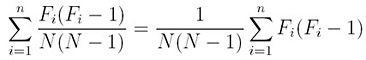Therefore, the index of coincidence is: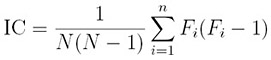Note that English has n = 26 letters.

### Example 1

Consider the plaintext of Hoare's quote discussed on the Kasiski's method page.

THERE ARETW OWAYS OFCON STRUC TINGA SOFTW AREDE SIGNO NEWAY
ISTOM AKEIT SOSIM PLETH ATTHE REARE OBVIO USLYN ODEFI CIENC
IESAN DTHEO THERW AYIST OMAKE ITSOC OMPLI CATED THATT HEREA
RENOO BVIOU SDEFI CIENC IESTH EFIRS TMETH ODISF ARMOR EDIFF
ICULT

The frequency count is as follows:

 A B C D E F G H I J K L M N O P Q R S T U V W X Y Z 15 2 9 7 27 8 2 9 20 0 2 4 5 10 19 2 0 12 15 22 4 2 5 0 4 0

The index of coincidence is 0.068101. The five highest frequency letters are E, T, I, O and A and S with 27, 22, 20, 19 and 15 occurrences, respectively. ♦

The following table shows the frequencies of the 26 English letters. The five most frequently used letters are E (13.11%), T (10.47%), A (8.15%), O (8.00%) and N (7.10%). The five least frequently used letters are Z (0.08%), Q (0.12%), J (0.13%), X (0.17%) and K (0.42%). Note that this table is generated from a sample of long English texts. Different samples yield slightly different results.

Frequency (%) of Letters in English Text
 A B C D E F G H I J K L M 8.15 1.44 2.76 3.79 13.11 2.92 1.99 5.26 6.35 0.13 0.42 3.39 2.54 N O P Q R S T U V W X Y Z 7.10 8.00 1.98 0.12 6.83 6.10 10.47 2.46 0.92 1.54 0.17 1.98 0.08

Given the frequency values as shown in the table above, it is not difficult to calculate the index of coincidence of English ICEnglish. Suppose the text has length N and the percentage of letter ai is pi. More precisely, p1 is the probability to have an A (i.e., pp = 8.15% = 0.0815), p2 is the probability to have a B (i.e., p2 = 1.44% = 0.0144), etc. The number of occurrences of the i-th letter is simply Fi = pi × N. Thererfore, we haveIf N is large enough, (piN-1)/(N-1) is approximately pi (e.g., the limit of this term as N approaches infinity), and we have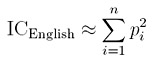Plugging the values in the English frequency table above we have ICEnglish = 0.0686\$. Note that the IC computed in Example 1 is 0.066332, which is close to the ICEnglish of English text.

What if the text is a randomly generated string? In this case, the frequency of each letter is approximately equal to pi = 1/n, where n is the size of the alphabet. Therefore, the index of coincidence for randomly generated text ICRandom ≈ 1/n. Since English has 26 letters, n = 26 and ICRandom ≈ 1/26 = 0.038466.

### Example 2

The following is the ciphertext of Hoare's quote encrypted with the Vigenère cipher and an unknown keyword (Example 1 of Kasiski's Method):

VVQGY TVVVK ALURW FHQAC MMVLE HUCAT WFHHI PLXHV UWSCI GINCM
UHNHQ RMSUI MHWZO DXTNA EKVVQ GYTVV QPHXI NWCAB ASYYM TKSZR
CXWRP RFWYH XYGFI PSBWK QAMZY BXJQQ ABJEM TCHQS NAEKV VQGYT
VVPCA QPBSL URQUC VMVPQ UTMML VHWDH NFIKJ CPXMY EIOCD TXBJW
KQGAN

The frequency count is shown below:

 A B C D E F G H I J K L M 11 6 11 3 5 5 6 13 8 4 7 5 12 N O P Q R S T U V W X Y Z 7 2 8 14 6 7 9 8 18 10 8 9 3

The IC reduces to 0.041989 because this is neither a plain English text nor random. ♦

### Example 3

The following is the ciphertext of the same quote of Hoare by shifting the plaintext to the right three positions (i.e., encrypted with a mono-alphabetic cipher):

WKHUH DUHWZ RZDBV RIFRQ VWUXF WLQJD VRIWZ DUHGH VLJQR QHZDB
LVWRP DNHLW VRVLP SOHWK DWWKH UHDUH REYLR XVOBQ RGHIL FLHQF
LHVDQ GWKHR WKHUZ DBLVW RPDNH LWVRF RPSOL FDWHG WKDWW KHUHD
UHQRR EYLRX VGHIL FLHQF LHVWK HILUV WPHWK RGLVI DUPRU HGLII
LFXOW

The frequency count is shown below:

 A B C D E F G H I J K L M 0 4 0 15 2 9 7 27 8 2 9 20 0 N O P Q R S T U V W X Y Z 2 4 5 10 19 2 0 12 15 22 4 2 5

Compared these values with those obtained in Example 1 for the same plaintext, we find that the table is also shifted to the right 3 positions. This is not a surprising result. If letter a appears n times in the plaintext and is shift to the left or right k positions to become the letter b = (a+k mod 26) in the ciphertext, then b also appears n times. Therefore, the IC should be the same as the one computed in Example 1. Indeed, the result is exactly 0.66332. ♦

Since ICRandom is the smallest IC we can get as the text is random, most if not all English texts should have an IC between ICRandom = 0.038466 and ICEnglish = 0.0686. A text whose IC is close to ICEnglish indicates that it is likely a valid English text or an English text encrypted with a mono-alphabetic cipher.

## References

• [CLARK1977] Clark, The Man Who Broke Purple, Little, Brown and Company, 1977.
• [FRIEDMAN1996] William F. Friedman, The Index of Coincidence and Its Applications in Cryptanalysis, Aegean Park Press, 1996. (This book was originally published in 1922 as Riverbank Publication No. 22, Riverbank Laboratories, Geneva, Illinois. It is also available from George C. Marshall Foundation here.)
• [KAHN1967] David Kahn, The Code Breakers, Macmillan, 1967. (A revised and updated edition was published by Scriber in 1996.)
• [KULLBACK1976] Solomon Kullback, Statistical Methods in Cryptanalysis, Aegean Park Press, 1976. (This book was originally published in 1938 by the Government Printing Office.)